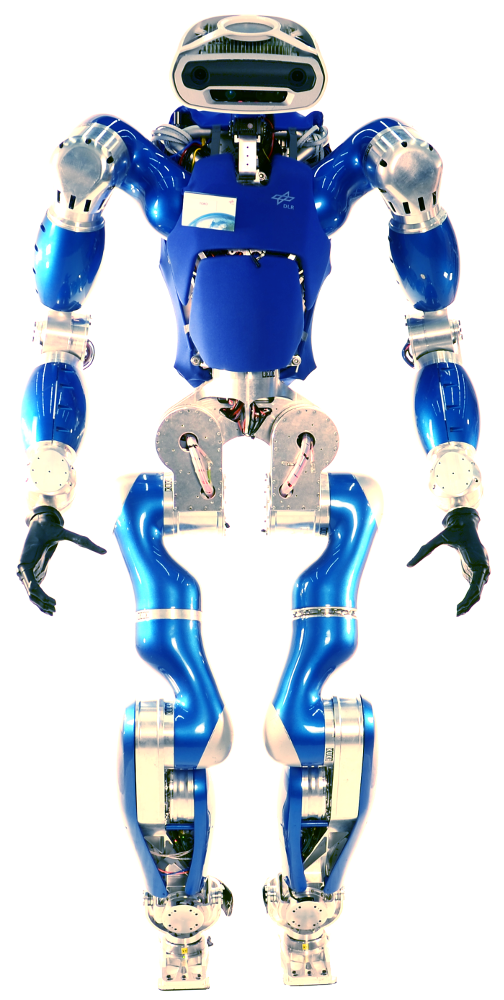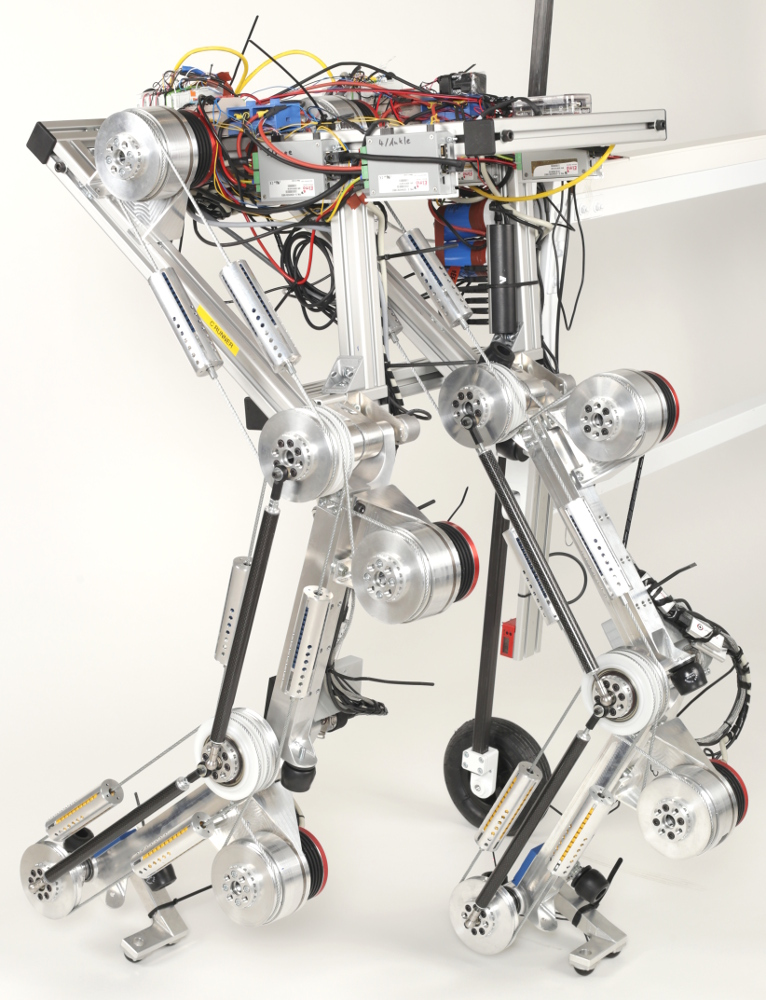# Walking models

## Small Model

#### Unilaterality#### Center of Pressure#### Friction Cone## Large Model

$$\boldsymbol{M}(\boldsymbol{y}) \boldsymbol{\ddot{y}} + \boldsymbol{C}(\boldsymbol{y},\boldsymbol{\dot{y}}) \boldsymbol{\dot{y}} + \boldsymbol{g}(\boldsymbol{y}) = \boldsymbol{S}^T \boldsymbol{\tau} + \sum_{i=0}^{N_c} \boldsymbol{J}^T_{c,i} \boldsymbol{W}_{c,i}$$

#### Added Constraints

• Joint Torques
• Joint velocities

#### Actuator dynamics (Series-Elastic Robot):

$$\boldsymbol{M} \boldsymbol{\ddot{q}} = \boldsymbol{\tau} - \boldsymbol{\tau}_{ext} \qquad \boldsymbol{\tau} = \boldsymbol{K} ( \boldsymbol{\theta} - \boldsymbol{q}) \qquad \boldsymbol{B} \boldsymbol{\ddot{\theta}} = \boldsymbol{\tau}_m - \boldsymbol{\tau}$$

# Optimal Control: Trajectory generation for walking

Human like gait anyone?

### 1. Parameterize

• All states: $$\boldsymbol{y} = f_\text{y}(\boldsymbol{p},t)$$
• Internal and External Forces $$\begin{bmatrix} \boldsymbol{\tau} \\ \boldsymbol{W}_\text{C,i} \end{bmatrix} = f_\tau(\boldsymbol{p},t)$$

### 2. Ensure physics:

$\boldsymbol{M}(\boldsymbol{y}) \boldsymbol{\ddot{y}} + \boldsymbol{C}(\boldsymbol{y},\boldsymbol{\dot{y}}) \boldsymbol{\dot{y}} + \boldsymbol{g}(\boldsymbol{y}) = \boldsymbol{S}^T \boldsymbol{\tau} + \sum_{i=0}^{N_c} \boldsymbol{J}^T_{c,i} \boldsymbol{W}_{c,i}$
$d_\mathrm{C,i,N} \cdot F_{C,i,N} + | \dot{d}_\mathrm{C,i,T} | \cdot F_\mathrm{C,i,N} = 0$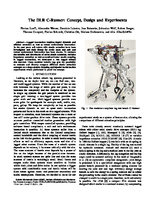IROS 2017: Generation of Locomotion Trajectories for Series Elastic and Viscoelastic Bipedal Robots A Werner, W Turlej and C Ott

# Dimensionality of walkingSimplified task space for bipedal locomotionProposed approach to use off-line computed trajectories in a feedback loop

# Generalization of Trajectories

1. sample task space in all dimensions of $k$
2. compute $p$ for all $k$ with optimization method
3. Ensure generate dataset of data is smoothResult structure for $\Gamma_{\tau}$
Generalize Dataset:
Using Gaussian Processes RegressionStill quite high sample density required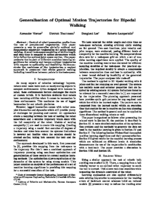IROS 2015: Generalization of optimal motion trajectories for bipedal walking - A Werner, D Trautmann, D Lee, R Lampariello

# CRunner Walking Robustly### Conclusions

• Robust robotics is fun
• Control of elastic systems is challenging
• Switching from impedance control to force control can be difficult
• Current Performance limits: Base state measurements
• A torque interface can still be provided
Accepted for Humanoid 2017: Optimal and Robust Walking using Intrinsic Properties of a Series-Elastic Robot - A Werner, B Henze, F Loeffl, C Ott

# Part 2: Perception and Step Planning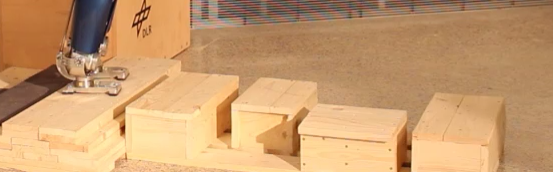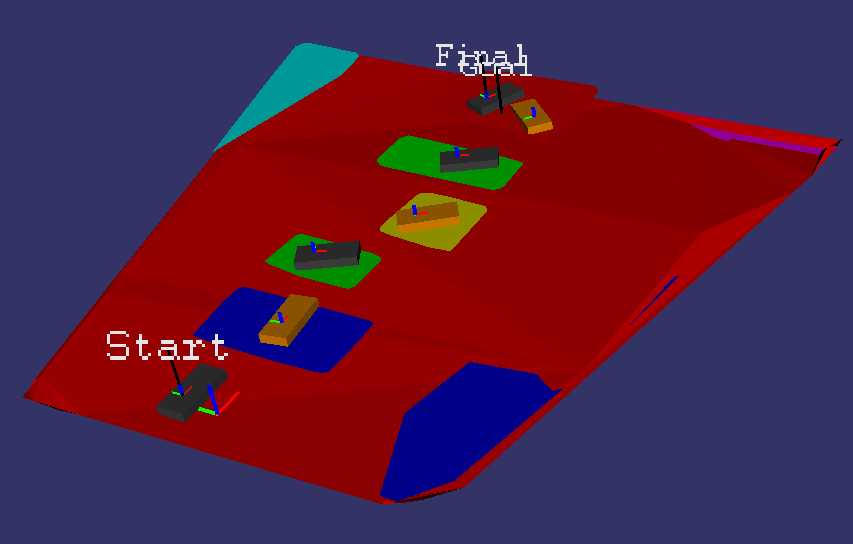IROS 2016: Multi-contact planning and control for a torque-controlled humanoid robot - Alexander Werner, Bernd Henze, Diego A Rodriguez, Jonathan Gabaret, Oliver Porges, Máximo A Roa

## Perception workflow

• Capture Image through RGBD Camera
• Cluster flat surfaces (steps)
• Compute convex hulls for each surface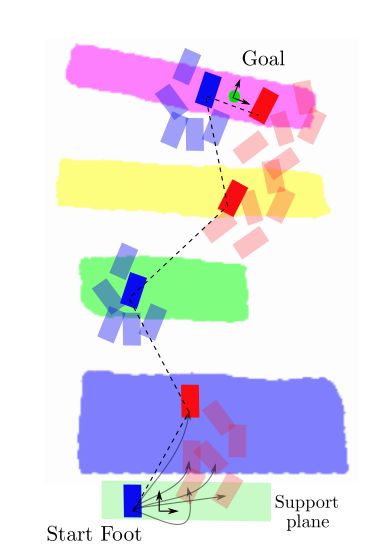## Step Planning

• Generated static stepping capabilities via "Posture Generator"
• Define criterion for foot-surface match
• Plan steps like A*

## Conclusions from Experiments

• Precision matters, errors accumulate
Perception: 2-3cm, Control: 1cm
• Accept partial contact of the foot
• Low Contact Stiffness makes balancing on one foot hard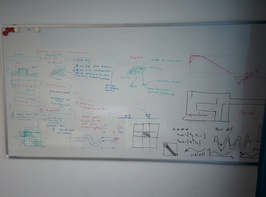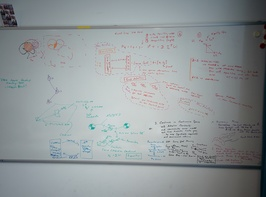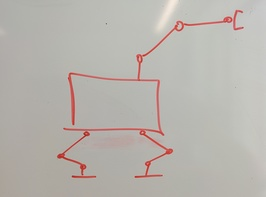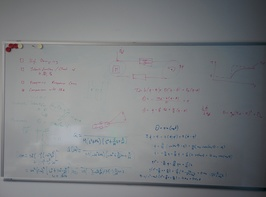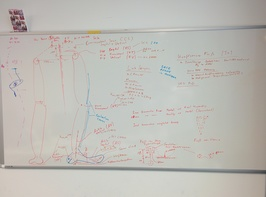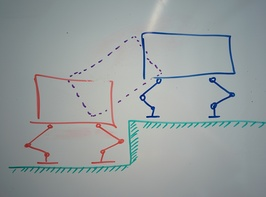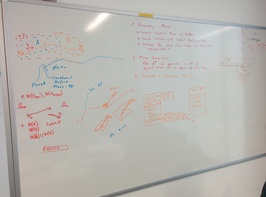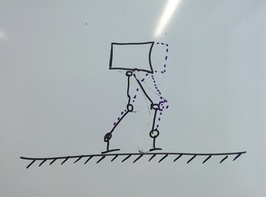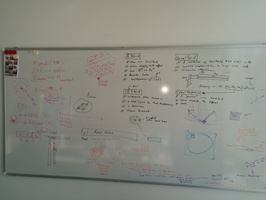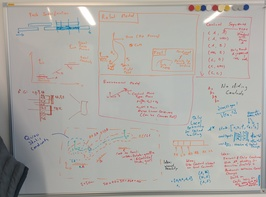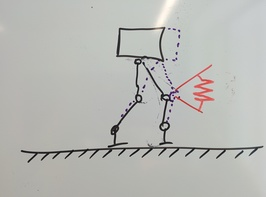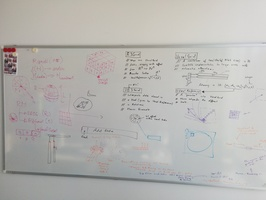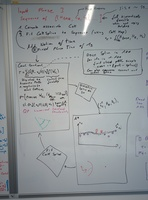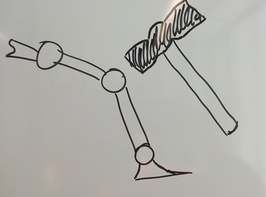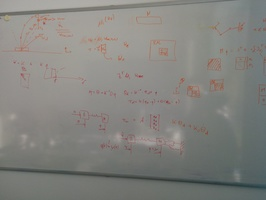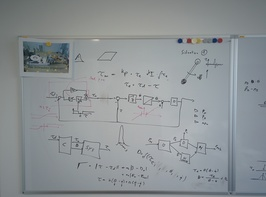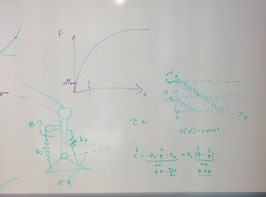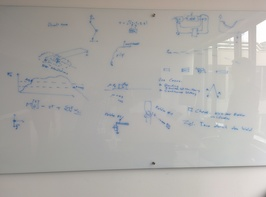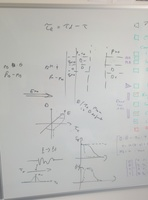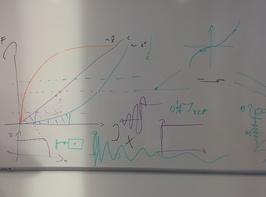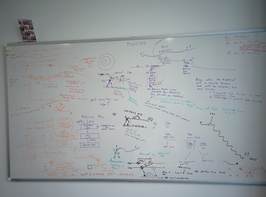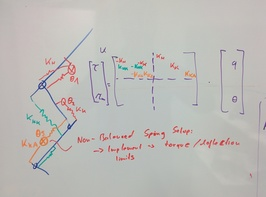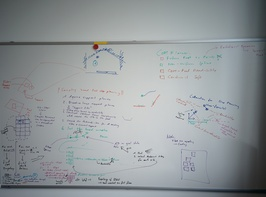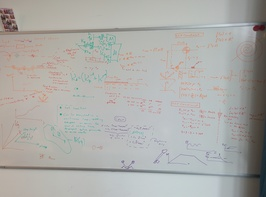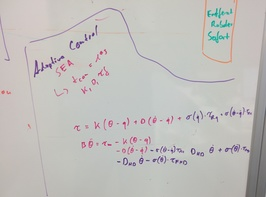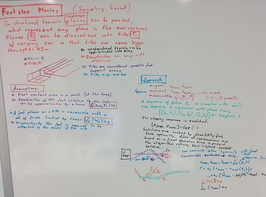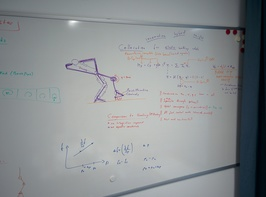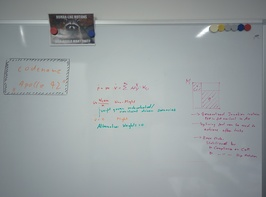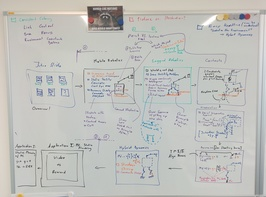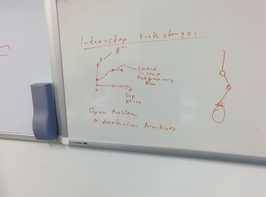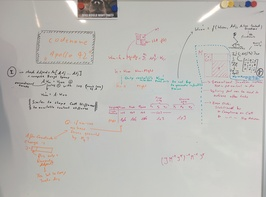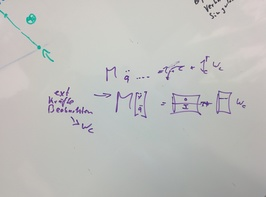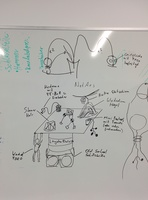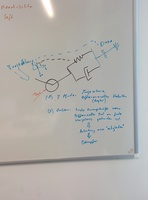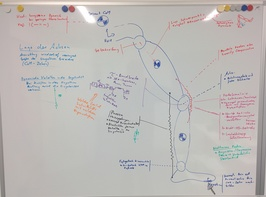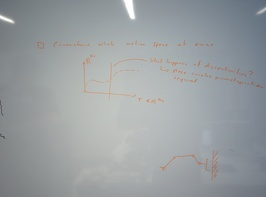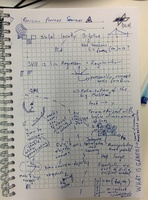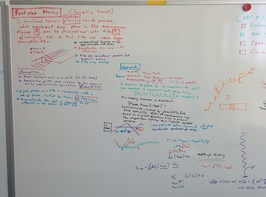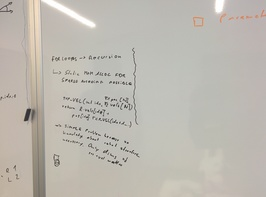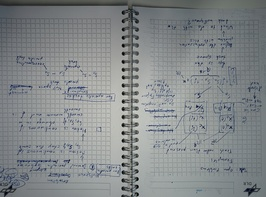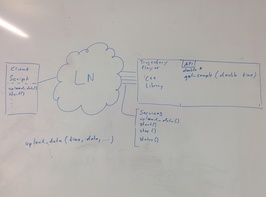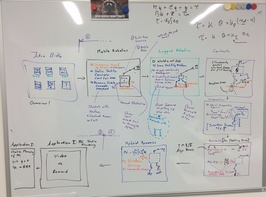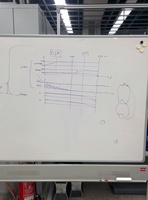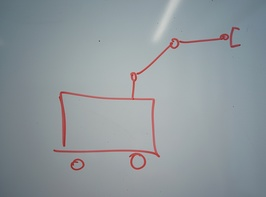# Questions